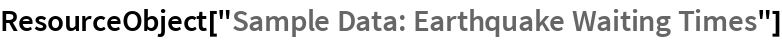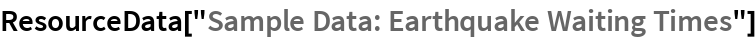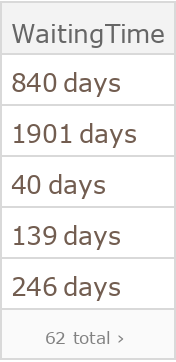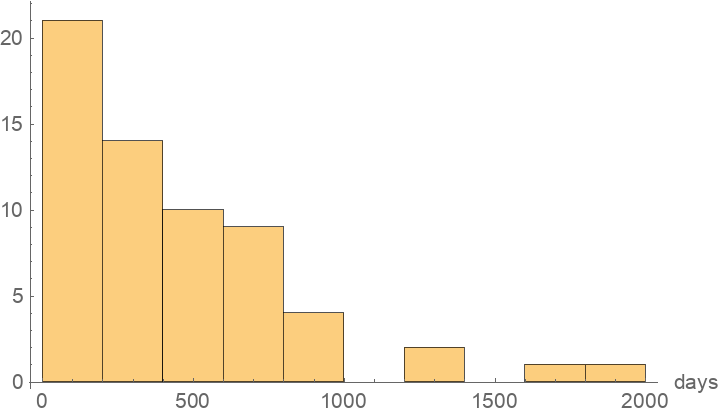# Sample Data: Earthquake Waiting Times

Earthquake waiting times

Originator: The Open University

## Examples

### Basic Examples

Retrieve the ResourceObject:

 In:=Out=View the data:

 In:=Out=### Visualization

Plot the distribution of times:

 In:=Out=Wolfram Research, "Sample Data: Earthquake Waiting Times" from the Wolfram Data Repository (2016) https://doi.org/10.24097/wolfram.50105.data